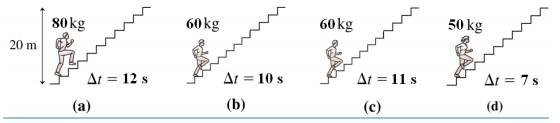# Problem: 1) Four students run up the stairs in the time shown. Rank in order, from largest to smallest, the mechanical work done by each student.2)Four students run up the stairs in the time shown. Rank in order, from largest to smallest, their power outputs Pa to Pd.

###### FREE Expert Solution

(1)

Work done against gravity:

$\overline{){\mathbf{W}}{\mathbf{=}}{\mathbf{m}}{\mathbf{g}}{\mathbf{h}}}$

Wa = magh = (80)(10)(20) = 16000J

Wb = mbgh = (60)(10)(20) = 12000J

Wc = mcgh = (60)(10)(20) = 12000J

89% (21 ratings)###### Problem Details1) Four students run up the stairs in the time shown. Rank in order, from largest to smallest, the mechanical work done by each student.

2)Four students run up the stairs in the time shown. Rank in order, from largest to smallest, their power outputs Pa to Pd.